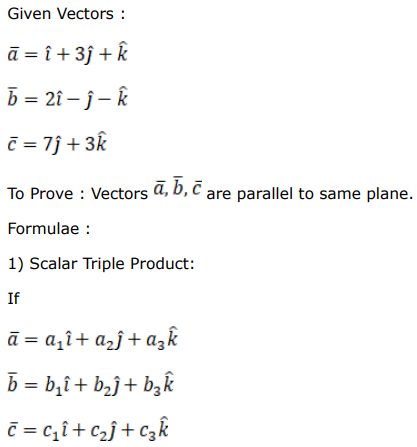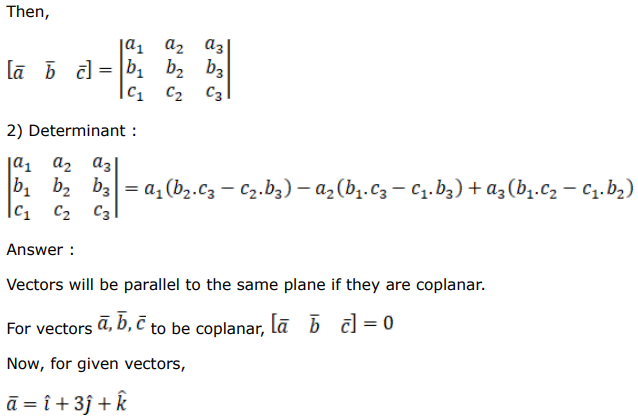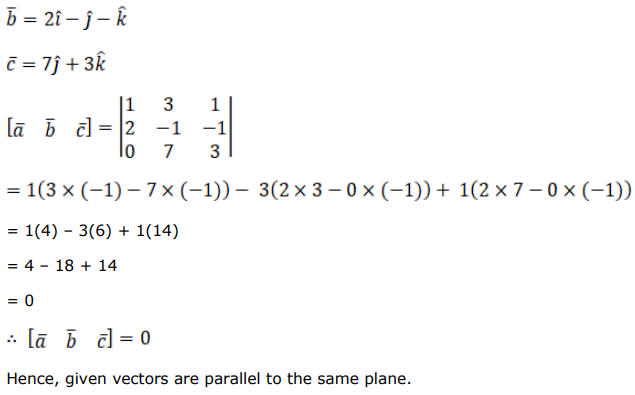# Solve this followingQuestion:

Show that the vectors $\overrightarrow{\mathrm{a}}=(\hat{\mathrm{i}}+3 \hat{\mathrm{j}}+\hat{\mathrm{k}}), \overrightarrow{\mathrm{b}}=(2 \hat{\mathrm{i}}-\hat{\mathrm{j}}-\hat{\mathrm{k}})$ and $\vec{c}=(7 \hat{j}+3 \hat{k})$ are parallel to the same plane.

\{HINT: Show that $[\overrightarrow{\mathrm{a}} \overrightarrow{\mathrm{b}} \overrightarrow{\mathrm{c}}]=0_{\text {\} }}$

Solution: